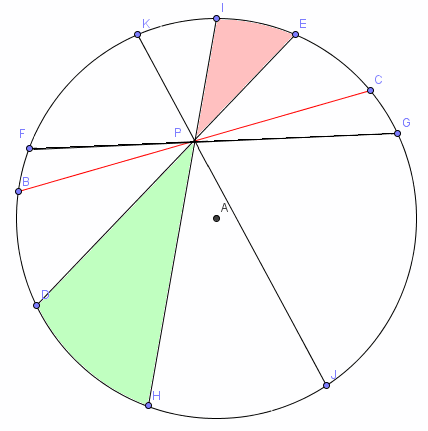Search IntMath
Close

450+ Math Lessons written by Math Professors and Teachers

5 Million+ Students Helped Each Year

1200+ Articles Written by Math Educators and Enthusiasts

Simplifying and Teaching Math for Over 23 Years

# Fair slicing of the pizza - using math

By Murray Bourne, 14 Dec 2009

Here's the situation. You order a pizza and the guy who cuts it for you has something in his eye and does a pretty bad job.

His first cut (BC) misses the center (A) and subsequent cuts are unevenly spaced, but at least he manages to get the cuts all passing through a single point (P).The question is - if 2 people are eating the pizza and they take alternate pieces, will they eat the same amount or will one of them get more?

It turns out there is some interesting math behind this problem.

You can read some of the solutions in The perfect way to slice a pizza, by Stephen Ornes of The New Scientist (requires a subscription)

Be the first to comment below.

### Comment Preview

HTML: You can use simple tags like <b>, <a href="...">, etc.

To enter math, you can can either:

1. Use simple calculator-like input in the following format (surround your math in backticks, or qq on tablet or phone):
a^2 = sqrt(b^2 + c^2)
(See more on ASCIIMath syntax); or
2. Use simple LaTeX in the following format. Surround your math with $$ and $$.
$$\int g dx = \sqrt{\frac{a}{b}}$$
(This is standard simple LaTeX.)

NOTE: You can mix both types of math entry in your comment.